# JavaScript function are First-class citizen

## JavaScript function are First-class citizen

In this article, I am going to discuss the JavaScript function are First-class citizen with Examples. Please read our previous article where we discussed Async Iterators and Generators in JavaScript. In JavaScript, functions are treated as first-class objects or first-class citizens, because they have the properties and methods the same as other objects. They can do the following:

• A function can have its own properties and method
• We can store the function in a variable
• We can pass the function as a parameter to another function
• We can return the function from other function as a value

In JavaScript, functions are first-class citizens. In simple words, we can treat functions like values of other types as it allows flexibility. Let see the example of the above-described function operation

##### Example: Function definition and can store in a variable
```<html>
<title>JavaScript first class object-function definition and store in a variable example</title>
<body>
<script>
// Function definition and invocation
function showMsg(string) {
console.log(string);
}
showMsg("Hello"); // logs "Hello"

// Store in a variable
return a + b;
}

console.log(result);//300
</script>
</body>
</html>```

Output: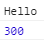##### Example: Pass the function as a parameter to another function
```<html>
<title>JavaScript first class object-pass the function as a parameter to another function example</title>
<body>
<script>
// Store in a variable
return a + b;
}

console.log(result);//return the function definition

function average(a, b, fnc) {
return fnc(a, b) / 2;
}

let avgResult = average(100, 200, add); );//passing function as arguement
console.log(avgResult);
</script>
</body>
</html>```

Output: 150

Since function value, we can pass a function as an argument to other function as well as we can return a function from other function

##### Example-1: Return a function from other function as a value
```<html>
<title>JavaScript first class object-Return a function from other function as a value example-1</title>
<body>
<script>
function comparisonBy(propName) {
return function (a, b) {
let x = a[propName],
y = b[propName];

if (x > y) {
return 1;
} else if (x < y) {
return -1
} else {
return 0;
}
}
}

let products = [
{ name: 'HP Laptop', price: 90000 },
{ name: 'Dell Laptop', price: 850000 },
{ name: 'Acer Laptop', price: 70000 }
];

console.log('Products sorted by name:');
products.sort(comparisonBy('name'));

console.table(products);

console.log('Products sorted by price:');
products.sort(comparisonBy('price'));

console.table(products);
</script>
</body>
</html>```

Output: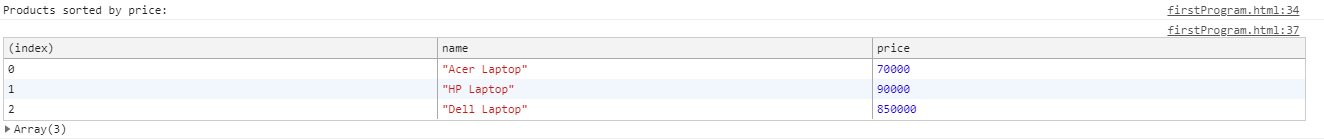In the above example, we have written a function comparisonBy() to compare two objects with the specified property. These objects are nothing but the array of product objects where each object contains the name and price property. And we are sorting the array by calling the sort() method

##### Example-2:
```<html>
<title>JavaScript first class object-Return a function from other function as a value example-2</title>
<body>
<script>
// Function definition and invocation
function speak(string) {
console.log(string);
}
speak("Hello! speak");                     // logs "Hello! speak"

// Store in a variable
var talk = speak;
talk("Hi! talk");                         // logs "Hi! talk"

// Pass as an argument to a function
// Return from a function
function functionReturner(fn) {
return fn;
}
var chat = functionReturner(talk);
chat("Good Morning! chat");               // logs "Good Morning! chat"
</script>
</body>
</html>```

Output:In the above example, we have created functionReturner() which does is accepts the function as an argument and returns it. And when we pass talk as an argument to functionReturner() function and then assign its return value into the variable chat, the same functionality happens with talk and speak like functionReturner() does.

##### Example: Function can have its own properties and method
```<html>
<title>JavaScript first class object-function can have its own properties and method example</title>
<body>
<script>
// Function definition and invocation
function speak(string) {
console.log(string);
}
speak("Hello! speak");                     // logs "Hello! speak"

// Store in a variable
var talk = speak;
talk("Hi! talk");                         // logs "Hi! talk"

// Owns properties
talk.myProperty = "start the conversation";
console.log(talk.myProperty);       // logs "start the conversation"
</script>
</body>
</html>```

Output:In the above example, we have assigned to function talk a property called myProperty with value start the conversation store in it. It shows that just like other objects, we can directly access the property of a function as we did in the example.

##### Higher-Order Functions

A higher-order function means the function which is at the top and being able to do other work on functions. In other words, a higher-order function is a function that either has a function as an argument or return a function.

The example of higher-order functions are built-in functions such as map, filter, and reduce that commonly use every day to manipulate JavaScript objects and data structures. The built-in function is the function that is provided by JavaScript itself.

These built-in functions perform a kind of work such as iteration or transformation. Most of the time, we pass these built-in functions with anonymous functions as callback arguments, but we can also pass in existing functions.

##### Examples of higher-order functions are map, filter, and reduce.

Like the below example:

##### Example: JavaScript high-order function example
```<html>
<title>JavaScript high-order function example</title>
<body>
<script>
var myNums = [1, 2, 3, 4, 5];

function doubleNum(num) {
return num * 2;
}

// Built-in Array.prototype.map function, using anonymous function argument
var doubledNums = myNums.map(function (num) {
return num * 2;
});
console.log(doubledNums);           // logs "[2, 4, 6, 8, 10]"

// Built-in Array.prototype.map function, using named callback argument
var otherDoubledNums = myNums.map(doubleNum);
console.log(otherDoubledNums);      // logs "[2, 4, 6, 8, 10]"
</script>
</body>
</html>```

Output: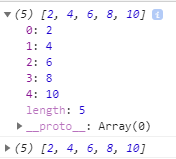In this above example, firstly, we have defined a variable myNums which contains an array of numbers. Later, we also define a function doubleNum which accepts a number as an argument/parameter and returns double the value of that number. We later then elaborate higher-order functions using built-in function Array.prototype.map, which iterates over an array, transforming each value and returning a new array with those values.

In the first example, we provide a map function with an anonymous function that doubles each value in the array. In the next subsequently function, we pass map our predefined function doubleNum, which it then uses in the transformation process. In both cases, we get the same result.

##### Example: JavaScript high-order function example-map, filter and reduce:
```<html>
<title>JavaScript high-order function example-map, filter and reduce</title>
<body>
<script>
console.log("Higher Order Functions");

// MAP
let arr = [1, 2, 3, 4, 5, 6];

//foreach function
arr.forEach((el) => {
console.log('foreach function without map function:', el ** 2)
})

//With IIFE=Immediately-invoked function expression i.e:()=>{} / ()=>
let mapfunc_square_IIFE = arr.map((el) => el ** 2);
console.log('map function with IIFE:', mapfunc_square_IIFE);

//With anonymous function
let mapfunc_square_anonymousfunc = arr.map(function (el) {
return el ** 2;
});

console.log('map with anonymous function:', mapfunc_square_anonymousfunc);

//Filter
let even = arr.filter(el => el % 2 == 0);

let evenSquares = arr.filter(el => el % 2 == 0).map(el => el ** 2);

console.log('even with filter', even);
console.log('evenSquares with filter and map:', evenSquares);

let users = [{ "user": "👩🏻‍💻" }, { "user": "👨🏾‍💻" }, { "user": "💃" }, { "user": "👨🏻‍🎓" }, { "user": "🧑🏻‍🏫" }, { "user": "🦸‍♂️" }, { "user": "🧟‍♂️" }];

let resultDetails = users.map(user => {
let mark = Math.random() * 100;
user.mark = mark;
return user;
})

console.log('resultDetails with map:', resultDetails, typeof (users));

let pass = resultDetails.filter(user => {
if (user.mark > 35) {
return user;
}
})

console.log('pass with filter:', pass);

// Reduce
let names = ['mumbai', 'delhi', 'goa'];
let red = names.reduce((acc, val) => {
let obj = {
name: val,
len: val.length,
}
acc.push(obj);
return acc;
}, []);

console.log('red with reduce:', red);

let sum = arr.reduce((acc, val) => {
acc += val;
return acc;
}, 0)

console.log('sum with reduce:', sum);
</script>
</body>
</html>```

Output: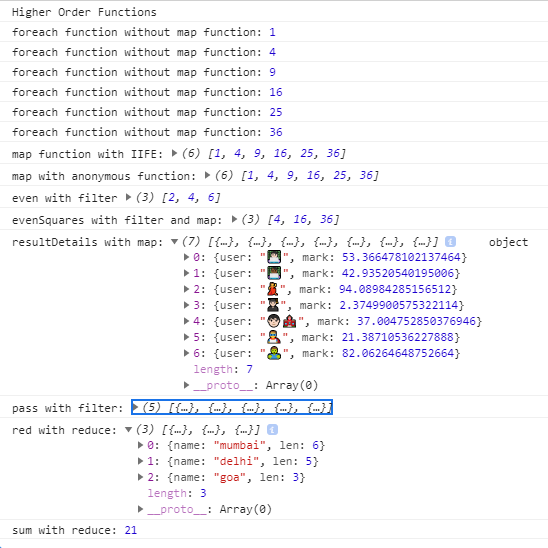In the above snippets, we have elaborated on the higher-order function such as map, filter, and reduce with IIFE and anonymous function. These functions help us to achieve the desired result by simplifying the code. We will learn more about map, filter and reduce in the map, filter and reduce chapter

##### Asynchronous functions in JavaScript:

The first-class function is used when an Asynchronous callback is mandatory. In other words when we want some tasks to be executed without waiting for other processes to complete. This can be used while downloading/uploading files from/to the server.

##### The example of asynchronous function is built-in function setTimeout() function.

We can simulate the asynchronous behavior by delaying the function call using the build-in function setTimeout, which in turn accepts the callback and a delay as per the time provided to it. We will gather more info about callback function in the callback function. Let see the example of it.

##### Example: Asynchronous function with setTimeout
```<html>
<title>JavaScript high-order function example-asynchronous function with setTimeout</title>
<body>
<script>
console.log("JavaScript high-order function example-asynchronous function with setTimeout")
function speak(string) {
console.log("speak=",string);
}

var delayedFunction = function (fn) {
return function (val, delay) {
setTimeout(function () {
fn(val);
}, delay);
};
};

var delayedSpeak = delayedFunction(speak);
delayedSpeak("I'm delayed speak function", 2000);// logs "I'm delayed speak function" after a 2 second delay
</script>
</body>
</html>```

Output: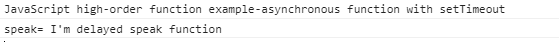In this above snippet, on line var delayedFunction = function (fn) where we define a new higher-order function called delayedFunction, which accepts a function as an argument and then returns a new function, which accepts a value and a delay. The newly created function uses setTimeout to wait for delay length (time in seconds) before executing the actual function we provided to delayedFunction with the provided value as its argument. We later, call delayedFunction on the second last line of the script, passing in our speak function, to create a new function called delayedSpeak. Now we can use speak with some delay!

Here we have not only used first-class functions, and higher-order functions, but also see how to asynchronously execute our code. The first-class function in JavaScript is used to make our code more flexible and shorter.